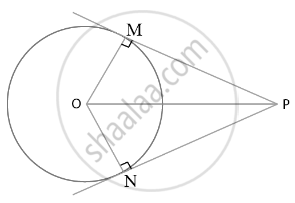# Prove that the Centre of a Circle Touching Two Intersecting Lines Lies on the Angle Bisector of the Lines . - Mathematics

Sum

Prove that the centre of a circle touching two intersecting lines lies on the angle bisector of the lines .

#### SolutionLet the lines be land l2.

Assume that O touches l₁ and l₂ at M and N,

We get,

OM = ON  ......(Radius of the circle)

Therefore,

From the centre ”O” of the circle, it has equal distance from l₁ and l₂.

In Δ OPM and OPN,

OM = ON  ......(Radius of the circle)

∠OMP = ∠ONP  ......(As, Radius is perpendicular to its tangent)

OP = OP .....(Common sides)

Therefore,

ΔOPM = ΔOPN ......(SSS congruence rule)

By C.P.C.T,

∠MPO = ∠NPO

So, l bisects ∠MPN.

Therefore, O lies on the bisector of the angle between l₁ and l₂ .

Hence, we prove that the centre of a circle touching two intersecting lines lies on the angle bisector of the lines.

Concept: Concept of Circle - Centre, Radius, Diameter, Arc, Sector, Chord, Segment, Semicircle, Circumference, Interior and Exterior, Concentric Circles
Is there an error in this question or solution?

#### APPEARS IN

NCERT Mathematics Exemplar Class 10
Chapter 9 Circles
Exercise 9.3 | Q 4 | Page 107
RD Sharma Class 10 Maths
Chapter 8 Circles
Exercise 8.2 | Q 49 | Page 41
Share